Roman Numerals 5
Set 5 (10 Questions)
Students learn the value of the various Roman Numerals. Students compare the value of Roman and standard numerals. Students have access to links about the historical use of Roman Numerals. Again, there are larger values than sets 1, 2, 3 & 4.
From Mr. Anker Tests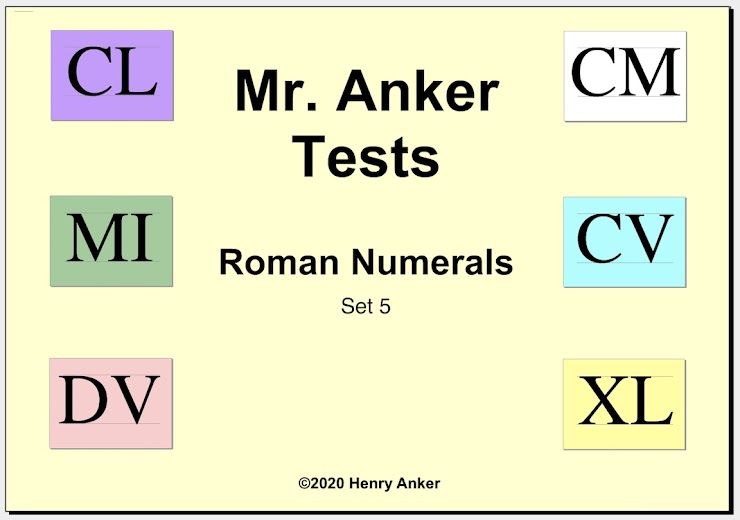Roman Numerals Help Slide 1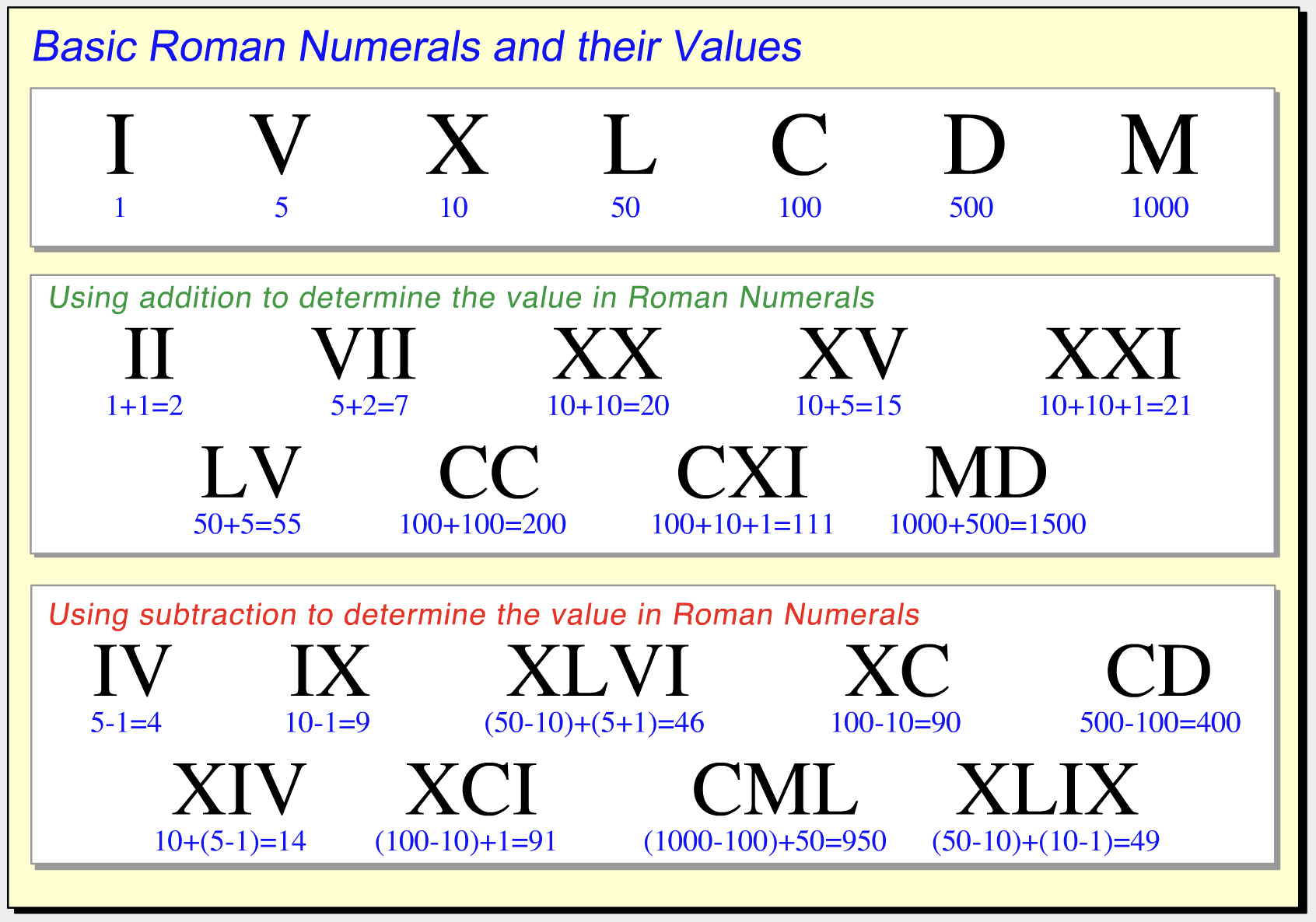This outstanding video can be found on Socratica Math
Roman Numerals Help Slide 2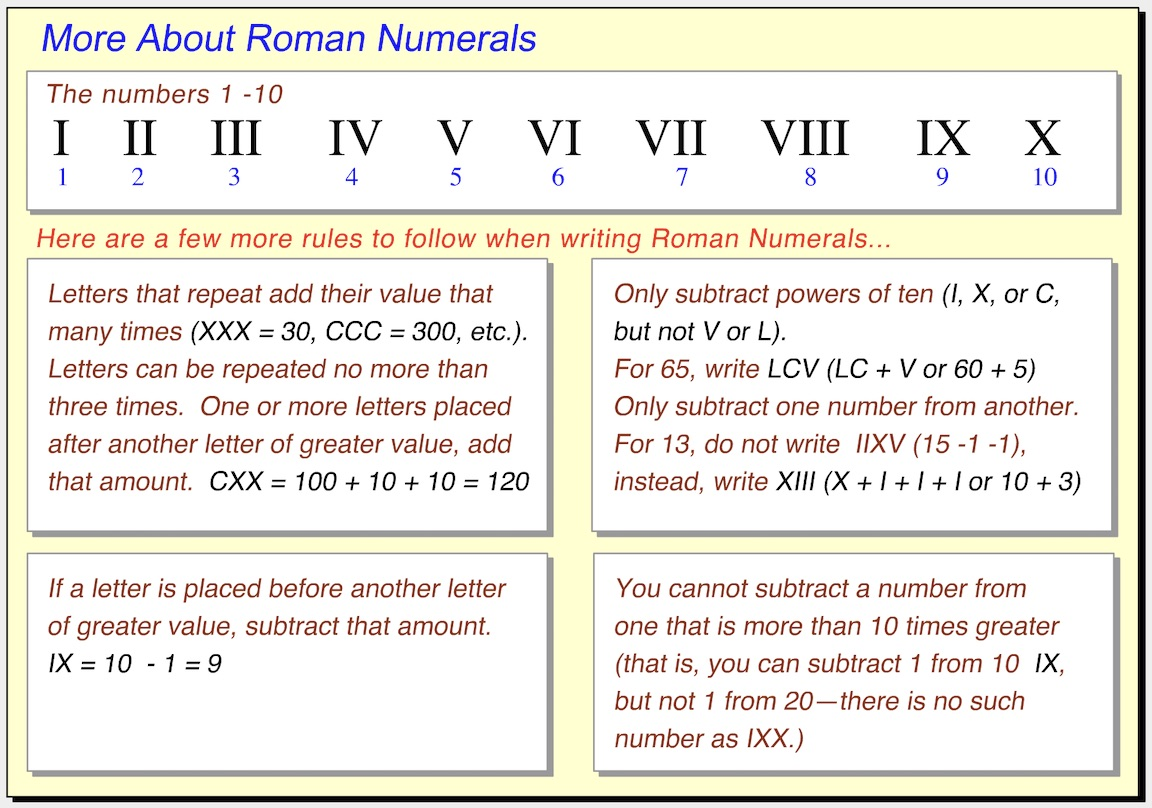1.  The Roman Numeral IC is ____________ the Roman Numeral CI. *
1 point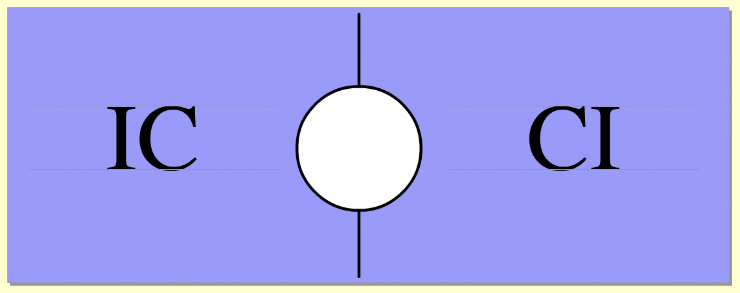2.  Match the standard numeral to this Roman Numeral. *
1 point3.  The standard numeral 53 is ____________  the Roman Numeral LII. *
1 point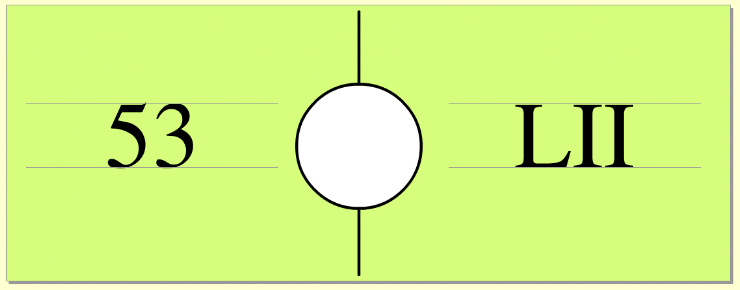4.  Match the standard numeral to this Roman Numeral. *
1 point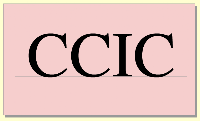5.  The Roman Numeral CLVII is ____________ the standard numeral 150. *
1 point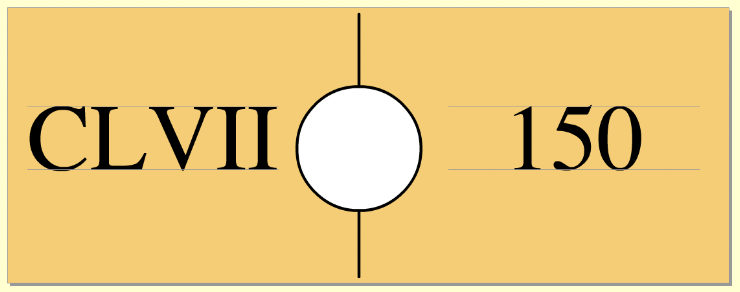6.  Match the Roman Numeral to this standard numeral. *
1 point7.  The Roman Numeral DL is ____________ the standard numeral 550. *
1 point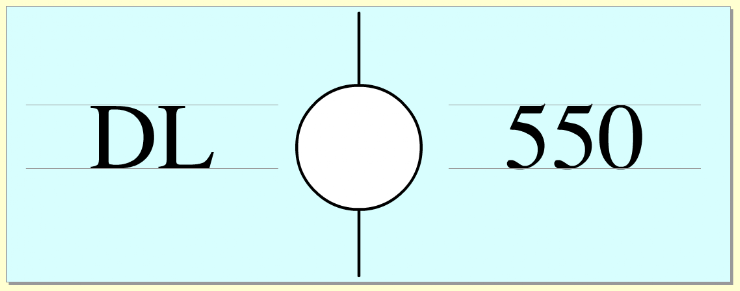8.  Match the standard numeral to this Roman Numeral. *
1 point9.  Enter, by typing, the standard numeral for this Roman Numeral. *
1 point10.  Enter, by typing, the standard numeral for this Roman Numeral. *
1 pointSubmit
Clear form